# Algebra 1 : How to find the sale price

## Example Questions

← Previous 1 3 4 5

### Example Question #1 : How To Find The Sale Price

A girl in County A spent $75 before a 7.25% sales tax and a girl in County B spent$70 before an 8% sales tax. How much more money did the girl from County A spend than the girl from County B after sales tax was applied? Round to the nearest hundredth.

$4.84$1.63

$2.63$5.84

$16.25 Correct answer:$4.84

Explanation:

County A: Multiply the price by the sales tax to find out how much money the sales tax will add. Remember to convert percent to decimal!

$75 * 0.0725 =$5.4375

Add the original price and the sales tax.

$75 +$5.4375 = $80.4375 County B: Multiply the price by the sales tax to find out how much money the sales tax will add. Remember to convert percent to decimal!$70 * 0.08 = $5.6 Add the original price and the sales tax.$70 + $5.6 =$75.6

Then take the difference.

80.4375 – 75.6 = 4.8375

Round to the nearest hundredth: $4.84 ### Example Question #1 : How To Find The Sale Price Mary was shopping for a new dress, and found one she liked for$48. Better yet, it was 25% off. How much did Mary pay for the dress?

$23$12

$36$30

$40 Correct answer:$36

Explanation:

To find the sale price we can set up a proportion. 25/100 = x/48. We keep the 100 and 48 both on the bottom of the fraction since they represent the "whole". x represents the discount off the full price of the dress. To solve this proportion, we cross multiply, yielding 48 * 25 = 100x. Alternatively, if you notice that 25/100 simplifies to 1/4, we can use 1/4 in our proportion instead of 25/100, thus, 1/4 = x/48. Then, cross multiplying, we get 48 = 4x, so x = 12. Then we subtract this discount from the original price yielding 48 – 12 = 36; thus, she paid $36 for the dress. 25% = 25/100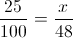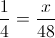(1) * (48) = (4) * (x) 48 = 4x (48)/4 = (4x)/4 12 = x The discount is$12.

$48 –$12 = $36 ### Example Question #3 : How To Find The Sale Price Robin sees that a jacket at the mall has been marked down 20% from an original price of$49.99. Taking into account the sales tax of 8%, which is applied after taking the discount, how much will Robin have left over if she brought $60 to the mall? Possible Answers: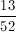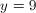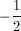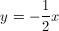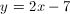Correct answer:Explanation: After the discount, she will pay for the remaining cost of the jacket. This cost will be equal to the percent remaining after the discount. 100% – 20% = 80% She will pay for 80% of the original cost. 80% = 0.80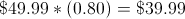Add in the sales tax. 8% = 0.08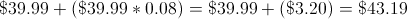Subtract the final price from Robin's budget of$60.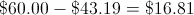There is a sale for 20% off on a sweater your grandma wants to buy you for christmas. The original price is $15.98. How much does the sweater cost with the discount? Possible Answers: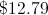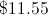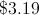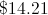Correct answer:Explanation: To find the answer, you must first convert the sales percentage to a decimal by moving the decimal left two places.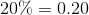Then, multiply the total cost by the decimal.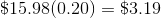This gives us the amount of the discount. To find the remaining cost, we subtract that number from the original cost to get the answer.### Example Question #1 : How To Find The Sale Price A shirt that originally cost$45 is on sale. The sale price is 15% off of the original price. What is the sale price?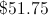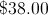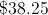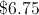Explanation:

First, calculate 15% of $45: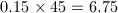Therefore, the discount is$6.75 off of the price of the shirt. To find the sale price, subtract the discount from the original price: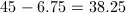### Example Question #1 : How To Find The Sale Price

An item is marked up by 25% and then marked down by 40%.  What percentage of the original price is the new price?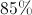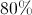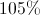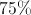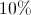Explanation:

The quickest way to do this problem is to realize that a "mark up" is done by adding that percentage to 100%.  For instance a 40% markup is performed by multiplying by 1.4.  A markdown is done by subtracting that percentage from 100%.  For instance, a 25% markdown is performed by multiplying by 0.75.  (Think of the latter case like this: it is 75% of its original price.)

Based on these remarks, the problem is easy.  While we do not know our price we know that the first markup gives us: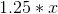To this, we now perform the mark down: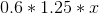Multiply out your coefficients (the numbers in the front):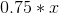This is the same thing as saying "75% of the original price ()."

The price of an item is $18 after a 25% discount. What was its original price? Possible Answers: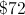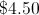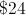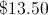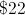Correct answer:Explanation: If something is discounted, it is really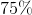of its original price. The question is thus asking, "of what is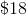?" This can be translated as: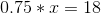Solve forby dividing both sides of the equation by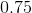: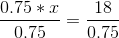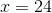Therefore, it was originally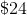. ### Example Question #8 : How To Find The Sale Price The price of an item is$25 after a 60% discount.  What was the original price?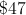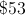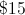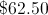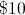Explanation:

When an item is marked down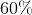, it isof its original price.  The question could be rephrased as "of what is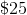?"  This could be rewritten as an equation rather simply: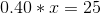Solve for x by dividing both sides by 0.40: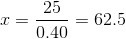.

### Example Question #9 : How To Find The Sale Price

At a department store, a high-end pair of jeans normally costs $170. This week, the store is having a sale and marked the jeans 20% off. However, Jane is an employee of the store and gets an additional 25% discount off the already discounted sale price. How much will Jane pay for the jeans? Possible Answers: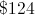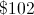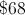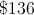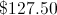Correct answer:Explanation: The first step is to find 20% of$170. This can be done easily by multiplying 170 by 0.2, yielding $34. We subtract 34 from 170 to get the initial sale price,$136. Next, we have to take 25% of that already discounted figure, and we do this by multiplying 136 by 0.25. Again, we happen to get $34, and after we subtract that number from 136 we get our final price,$102. The other answer choices are either the result of forgetting a step or of simple calculation errors.

### Example Question #10 : How To Find The Sale Price

Mr. Jones is trying to restrict his expenditures on groceries to 12% of his salary. He makes $756.00 weekly, and he lives in a state where the sales tax rate is 6.125% on groceries. Calculate the maximum price before sales tax of the groceries Mr. Jones can buy each week in order to stick to his self-imposed limit. Possible Answers: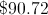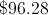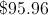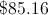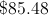Correct answer:Explanation: According to his rule, Mr. Jones can spend 12% of$756.00 weekly.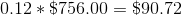This is the amount he can spend after sales tax.

Letbe the price of the groceries before the sales tax is figured in.

The sales tax paid is 6.125% of, or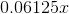, so the amount paid for the groceries is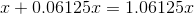.

Since Mr. Jones is limited to spending $90.72, this means that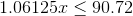. We can solve the inequality for.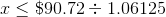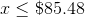The maximum amount Mr. Jones can spend before tax is$85.48.

← Previous 1 3 4 5